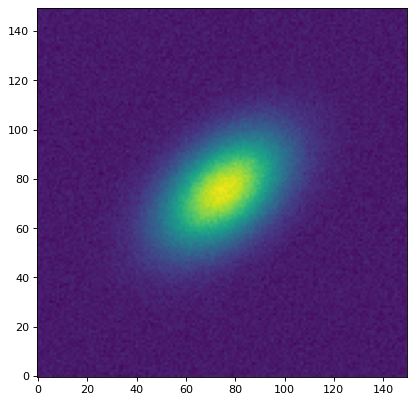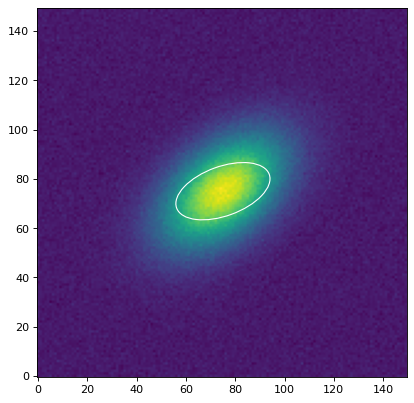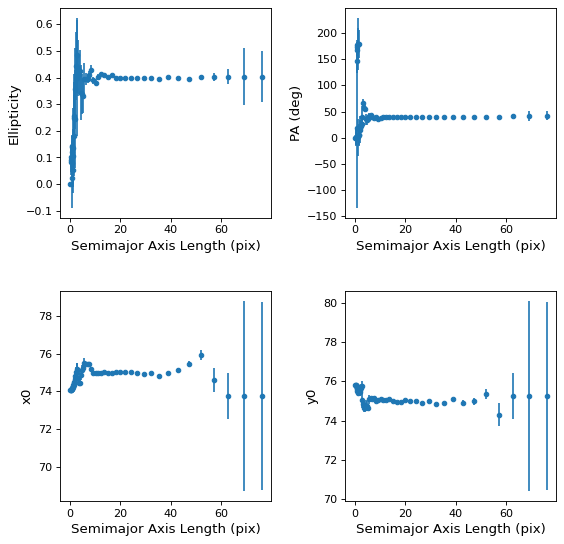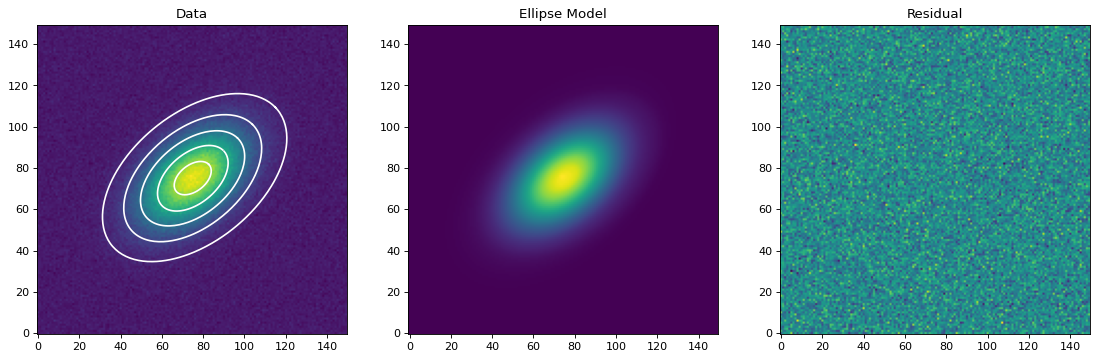# Elliptical Isophote Analysis (`photutils.isophote`)¶

## Introduction¶

The `isophote` package provides tools to fit elliptical isophotes to a galaxy image. The isophotes in the image are measured using an iterative method described by Jedrzejewski (1987; MNRAS 226, 747). See the documentation of the `Ellipse` class for details about the algorithm. Please also see the Isophote Frequently Asked Questions.

## Getting Started¶

For this example, let’s create a simple simulated galaxy image:

```>>> from astropy.modeling.models import Gaussian2D
>>> import numpy as np
>>> from photutils.datasets import make_noise_image

>>> g = Gaussian2D(100., 75, 75, 20, 12, theta=40. * np.pi / 180.)
>>> ny = nx = 150
>>> y, x = np.mgrid[0:ny, 0:nx]
>>> noise = make_noise_image((ny, nx), distribution='gaussian', mean=0.,
...                          stddev=2., seed=1234)
>>> data = g(x, y) + noise
```We must provide the elliptical isophote fitter with an initial ellipse to be fitted. This ellipse geometry is defined with the `EllipseGeometry` class. Here we’ll define an initial ellipse whose position angle is offset from the data:

```>>> from photutils.isophote import EllipseGeometry
>>> geometry = EllipseGeometry(x0=75, y0=75, sma=20, eps=0.5,
...                            pa=20. * np.pi / 180.)
```

Let’s show this initial ellipse guess:

```>>> import matplotlib.pyplot as plt
>>> from photutils.aperture import EllipticalAperture
>>> aper = EllipticalAperture((geometry.x0, geometry.y0), geometry.sma,
...                           geometry.sma * (1 - geometry.eps),
...                           geometry.pa)
>>> plt.imshow(data, origin='lower')
>>> aper.plot(color='white')
```Next, we create an instance of the `Ellipse` class, inputting the data to be fitted and the initial ellipse geometry object:

```>>> from photutils.isophote import Ellipse
>>> ellipse = Ellipse(data, geometry)
```

To perform the elliptical isophote fit, we run the `fit_image()` method:

```>>> isolist = ellipse.fit_image()
```

The result is a list of isophotes as an `IsophoteList` object, whose attributes are the fit values for each `Isophote` sorted by the semimajor axis length. Let’s print the fit position angles (radians):

```>>> print(isolist.pa)
[ 0.          0.16838914  0.18453378  0.20310945  0.22534975  0.25007781
0.28377499  0.32494582  0.38589202  0.40480013  0.39527698  0.38448771
0.40207495  0.40207495  0.28201524  0.28201524  0.19889817  0.1364335
0.1364335   0.13405719  0.17848892  0.25687327  0.35750355  0.64882699
0.72489435  0.91472008  0.94219702  0.87393299  0.82572916  0.7886367
0.75523282  0.7125274   0.70481612  0.7120097   0.71250791  0.69707669
0.7004807   0.70709823  0.69808124  0.68621341  0.69437566  0.70548293
0.70427021  0.69978326  0.70410887  0.69532744  0.69440413  0.70062534
0.68614488  0.7177538   0.7177538   0.7029571   0.7029571   0.7029571 ]
```

We can also show the isophote values as a table, which is again sorted by the semimajor axis length (`sma`):

```>>> print(isolist.to_table())
sma            intens        intens_err   ... flag niter stop_code
...
-------------- --------------- --------------- ... ---- ----- ---------
0.0   102.237692914             0.0 ...    0     0         0
0.534697261283   101.212218041 0.0280377938856 ...    0    10         0
0.588166987411   101.095404456  0.027821598428 ...    0    10         0
0.646983686152   100.971770355 0.0272405762608 ...    0    10         0
0.711682054767   100.842254551 0.0262991125932 ...    0    10         0
...             ...             ... ...  ...   ...       ...
51.874849202   3.44800874483 0.0881592058138 ...    0    50         2
57.0623341222   1.64031530995 0.0913122295433 ...    0    50         2
62.7685675344  0.692631010404 0.0786846787635 ...    0    32         0
69.0454242879  0.294659388337 0.0681758007533 ...    0     8         5
75.9499667166 0.0534892334515 0.0692483210903 ...    0     2         5
Length = 54 rows
```

Let’s plot the ellipticity, position angle, and the center x and y position as a function of the semimajor axis length:We can build an elliptical model image from the `IsophoteList` object using the `build_ellipse_model()` function ( NOTE: this function requires scipy):

```>>> from photutils.isophote import build_ellipse_model
>>> model_image = build_ellipse_model(data.shape, isolist)
>>> residual = data - model_image
```

Finally, let’s plot the original data, overplotted with some of the isophotes, the elliptical model image, and the residual image:Additional example notebooks showing examples with real data and advanced usage are available online:

## Reference/API¶

This subpackage contains tools for fitting elliptical isophotes to galaxy images.

### Functions¶

 `build_ellipse_model`(shape, isolist[, fill, …]) Build a model elliptical galaxy image from a list of isophotes. Compute the harmonic function value used to calculate the corrections for ellipse fitting. `fit_first_and_second_harmonics`(phi, intensities) Fit the first and second harmonic function values to a set of (angle, intensity) pairs. `fit_upper_harmonic`(phi, intensities, order) Fit upper harmonic function to a set of (angle, intensity) pairs.

### Classes¶

 `Ellipse`(image[, geometry, threshold]) Class to fit elliptical isophotes to a galaxy image. `EllipseFitter`(sample) Class to fit ellipses. `EllipseGeometry`(x0, y0, sma, eps, pa[, …]) Container class to store parameters for the geometry of an ellipse. `EllipseSample`(image, sma[, x0, y0, astep, …]) Class to sample image data along an elliptical path. `Isophote`(sample, niter, valid, stop_code) Container class to store the results of single isophote fit. `IsophoteList`(iso_list) Container class that provides the same attributes as the `Isophote` class, but for a list of isophotes.